# General recursive function

(diff) ← Older revision | Latest revision (diff) | Newer revision → (diff)
A partial recursive function that is defined for all values of its arguments. The concept of a general recursive function can also be defined independently of the concept of a partial recursive function as follows. The class of all general recursive functions is the smallest class of functions containing all primitive recursive functions (cf. Primitive recursive function) and closed under composition of functions and the least-number operator, under the condition that the latter is only applied to a functionwhen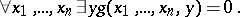However, the study of general recursive functions is usually carried out within the class of all partial recursive functions. This is related, in particular, to the fact that there is no number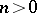for which there exists a general recursive function that is universal for the class of all-place general recursive functions.
All general recursive functions are explicitly definable in formal arithmetic (cf. Arithmetic, formal) in the sense that for any such functionan arithmetical formula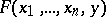can be constructed with the following property: For any natural numbers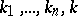, if, then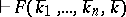; but if, then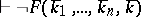, whereare the terms representing the numbers, and the symboldenotes derivability in the arithmetic calculus.